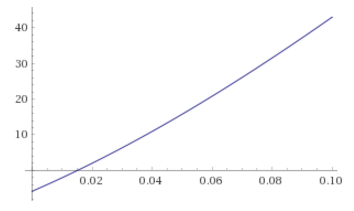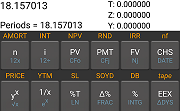### NEWTON-RAPHSON METHOD APPLIED TO FINDING INTEREST RATE ON A LOAN:

##### LOAN MATHEMATICS:

We take a loan out from the bank: the principal $$P$$ to be repaid in a fixed amount of time (let’s assume monthly payments $$n =360$$, corresponding to monthly payments for $$30$$ years) with interest compounded every month $$i = \text{nominal interest}/12$$. Every month the payment is $$R$$ (“rent”) dollars.

At time zero, we take the loan, and it starts accruing interest. After a month, the amount owed will be:

$P \; (1 +i)$

at which point we make the first payment. Now the balance will be left at

$\text{balance owed}= P \; (1 +i) - R$

on which interest will be accrued, so that by the end of the second month (and after making the second payment) the balance will be

\begin{align}\text{balance owed} &= \left [P\; (1 + i) - R\; \right] \;(1 + i) - R\\[2ex] &= P\;(1+i)^2-R(1+i)-R \end{align}

At the end of the third month the balance will be

\begin{align}\text{balance owed} &= \left[P\;(1+i)^2-R\;(1+i)-R\;\right]\;(1+i)-R\\[2ex] &= P\;(1+i)^3-R\;(1+i)^2-R\;(1+i)-R \end{align}

At the end of the $$n$$ payments, the balance will be zero

\begin{align} 0 &= P\;(1+i)^n-R\;(1+i)^{n-1}-R\;(1+i)^{n-2}-\;\cdots\;-R\;(1+i)^0\\[2ex] &=P\;(1+i)^n - R\;\frac{1-(1+i)^n}{1-(1+i)}\\[2ex] &=P\;(1+i)^n - R\;\frac{(1+i)^n-1}{(1+i)-1}\tag 1\\[2ex] &=P\;(1+i)^n - R\;\frac{(1+i)^n-1}{i} \end{align}

because $$-R\;(1+i)^{n-1}-R\;(1+i)^{n-2}-\;\cdots\;-R\;(1+i)^0$$ forms a geometrical series, and

$\sum_{k=0}^{n-1}a\;r^k=a\;\frac{1-r^n}{1-r}$

Rearranging,

\begin{align} R\;\frac{(1+i)^n-1}{i}&=P\;(1+i)^n\\[2ex] R\;\left((1+i)^n-1\right)&=P\;i\;(1+i)^n\\[2ex] R&=P\;i\;\frac{(1+i)^n}{\left((1+i)^n-1\right)}\tag 2 \end{align}

##### NEWTON-RAPHSON METHOD:

We want to find the zro values for Eq $$(1)$$, and we resort to the Newton-Raphson method, which solves for the $$x$$ value at which $$F(x)=0$$ based on a guess that this $$x$$ value is close to an $$a$$ value, wuch that

$F'(a) = \lim_{a\to x}\; \frac{F(x)-F(a)}{x-a}$

Assuming that the guess gets better and better, we can get rid of the $$\lim$$, and express this formula as

$F'(a) \approx \frac{F(x)-F(a)}{x-a}$

Keeping in mind that $$F(x)$$ is a zero,

$x\approx a -\frac{F(a)}{F'(a)}$

Since $$a$$ can actually be far from $$x$$ we update $$x$$ as

$x_{i+1} = x_i-\frac{F(x_i)}{F'(x_i)}$

Going back to equation $$(2),$$ and taking into consideration that $$i$$ is the variable to optimize,

$F(x) = \frac{P\;x\;(1+x)^n}{\left((1+x)^n-1\right)}-R$

Therefore,

\begin{align} F'(x)&=\frac{\partial \left(\frac{P x (x+1)^n}{(x+1)^n-1}-R\right)}{\partial x}\\[2ex] &=\frac{n P x (x+1)^{n-1}}{(x+1)^n-1}+\frac{P (x+1)^n}{(x+1)^n-1}-\frac{n P x (x+1)^{2 n-1}}{\left((x+1)^n-1\right)^2}\\[2ex] &=\frac{P (x+1)^{n-1} \left(x (x+1)^n+(x+1)^n-n x-x-1\right)}{\left((x+1)^n-1\right)^2} \end{align}

Let’s now give some values to the principal and the payment… Say we bought a new phone because we were eligible after a couple of years of using a the iFone 3.0. The new phone is the iFone 3.1, and costs $$P= \ 700$$. The salesman at AT&T tells you that you’ll pay $$R =\ 35$$ for $$n=24$$ months. What is the interest rate being charged?

$F(x) = \frac{700\;x\;(1+x)^{24}}{\left((1+x)^{24}-1\right)}-35$

$F'(x)=\frac{16800\, x (x+1)^{23}}{(x+1)^{24}-1}+\frac{P (x+1)^{24}}{(x+1)^{24}-1}-\frac{16800\, x (x+1)^{47}}{\left((x+1)^{24}-1\right)^2}$

Plotting $$F(x),$$would allow us to start the guessing game at $$0.02$$, which corresponds to a nominal annual interest of $$0.02 \times 12=24\%.$$ Otherwise we could just pretend that there is no compounding and guess $$\frac{R\times n}{P}=\frac{35\times 24}{700}= 1.2\implies i = 0.2/12= 0.016.$$

In this post there is a nice simplification that helps get a cleaner code expression, and a simpler derivative: instead of working with $$i=x,$$ we set up $$x=1+\frac{i}{12},$$ in which case, and going back to equation $$(1),$$

\begin{align} &P\;(1+i)^n = R\;\frac{(1+i)^n-1}{(1+i)-1}\\[2ex] &P\,x^n\,(x-1)=R\,(x^n-1)\\[2ex] &F(x)=P\,x^n\,(x-1)-R\,(x^n-1)\\[2ex] &F(x)=P\,x^{n+1}\,-(P+R)\,x^n+R \end{align}

a nice polynomial, which derivative is

$F'(x)=P\, (n+1)\, x^n-(P+R)\,n\,x^{n-1}$

And the R code,

interest = function(loan, pmt, n){
F =       function(x) (loan * x^(n+1) - (loan + pmt) * x^n + pmt)
F_prime = function(x) (loan * (n+1) * x^n - (loan + pmt) * n * x^(n-1))

guess = 1 + (((pmt * n / loan) - 1) / 12)

x = guess

while(abs(F(x)) > (1e-6)){
x = x -  F(x)/ F_prime(x)
print(x)
}
paste("Yearly interest: ", round((12 * (x - 1) * 100),3)," percent")
}

interest(700,35,24)
##  1.01529
##  1.015133
##  1.015131
##  "Yearly interest:  18.157  percent"

Double-checking with a financial calculator:

700   PV
0     FV
-35   PMT
24    n
i
g     12xOf course, this should also work out using the more convoluted formulas:

interest = function(loan, pmt, n){
F =       function(x) (loan * x * (1 + x)^n)/((1+x)^n - 1) - pmt
F_prime = function(x) (loan * (x+1)^(n-1) * (x*(x+1)^n + (x+1)^n-n*x-x-1)) / ((x+1)^n - 1)^2

guess = 1 + (((pmt * n / loan) - 1) / 12)

x = guess

while(abs(F(x)) > (1e-6)){
x = x -  F(x)/ F_prime(x)
print(x)
}
paste("Yearly interest: ", round((12 * x * 100),3)," percent")
}

interest(700,35,24)
##  0.04999943
##  0.01812481
##  0.01515984
##  0.01513085
##  0.01513084
##  "Yearly interest:  18.157  percent"